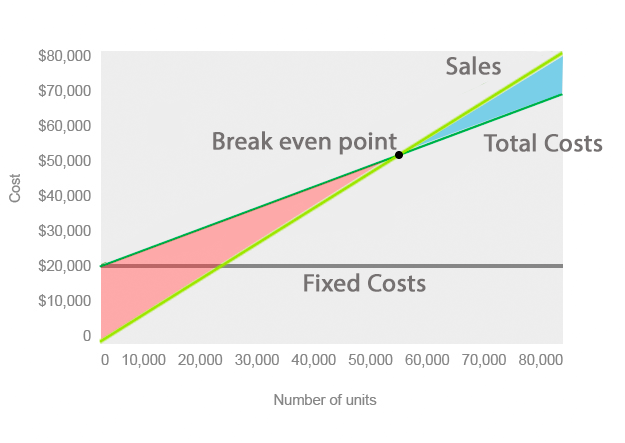# What is Cost Volume Profit Analysis (CVP)?

Definition: The cost volume profit analysis, commonly referred to as CVP, is a planning process that management uses to predict the future volume of activity, costs incurred, sales made, and profits received. In other words, it’s a mathematical equation that computes how changes in costs and sales will affect income in future periods.

## What Does Cost Volume Profit Analysis Mean?

The CVP analysis classifies all costs as either fixed or variable. Fixed costs are expenses that don’t fluctuate directly with the volume of units produced. These costs effectively remain constant. An example of a fixed cost is rent. It doesn’t matter how many units the assembly line produces. The rent expense will always be the same.

## Example

Variable costs, on the other hand, change with the levels of production. These costs include materials and labor that go into each unit produced. For example, a bike factory would classify bicycle tire costs as a variable cost. Every bike that is produced must have two tires. The more units produced, the more tire costs increase.The CVP analysis uses these two costs to plot out production levels and the income associated with each level. As production levels increase, the fixed costs become a smaller percentage of total income while variable costs remain a constant percentage. Cost accountants and management analyze these trends in an effort to predict what costs, sales, and profits the company will have in the future.

They also use cost volume profit analysis to calculate the break-even point in production processes and sales. The break-even point is drawn on the CVP graph where the sales, fixed costs, and variable costs’ lines all intersect. This is a key concept because it shows management that the revenue from a project will be able to cover all the costs associated with it. Using a variation of the CVP, management can calculate the break-even point in profits, units, and even dollars.